## What is Digital Electronics (ART301E)

The digital word comes from "digitus," which in Latin means "finger," since the most commonly used numbering systems are based entirely on the fingers of our hands. To count objects, the ancients and even us, used the fingers as a reference.

NOTA DO AUTOR

Note: this text is from an author's book published in 1987, but perfectly current.

FIM NOTA

The Romans themselves developed a system in which the main references were rightly due to full hands leading to larger quantities.

Thus, arriving at a full hand or 5 units, one would use another symbol, the V which means the hand with two open fingers. For 10 units, they had the X meaning two crossed hands, or two full hands (See Figure 1).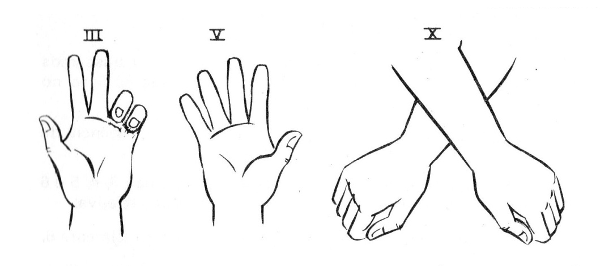Figure 1

See that our "decimal" system derives precisely from the fact that we have 10 fingers in our hands.

When we "fill" both hands, that is, we have an amount that is represented by 10 fingers, we have to use more than one sign other than the one we know from 0 to 9. We then use a signal to say "how many hands we have" and how many more units.

The number 27, for example, means that we have 2 hands (two tens) and 7 more.

Note that using this numbering system we can only represent discrete quantities, that is, integers.

We cannot "work" (yet) with broken quantities such as two and a half objects.

This differentiates the digital system from the analog.

Initially the reader should keep in mind that in the digital system, only integer quantities can be represented, which does not occur in an analog system in which any intermediate value between two units can be represented.

In fact, the digital system also admits this kind of representation, with some sacrifices, but this will only be seen much later.

The system we use, is usually the base 10, that is, we use 10 different digits to represent any quantity:

0 1 2 3 4 5 6 7 8 9

To represent an amount greater than 10, we use the same digits, but with a position in the number that happens to have a "weight."

This "weight" or relative value is always a power of 10, that is, it can be 10, 100, 1 000, 10 000, etc.

Thus, the number 3 456 has its figures 3, 4, 5 and 6 with different weights corresponding to its relative position:

The 6 has "weight 1" and should therefore really mean 6.

The 5 has "weight 10", that is, it represents 5 tens or 50.

The 4 has "weight 100", that is, it represents 4 hundreds or 400.

The 3 has "weight 1 000", that is, it represents 3 thousands or 3 000.

Summing up the values ??that each digit represents, we have the total value of the number 3 thousands, 4 hundreds, 5 tens and 6 units, or as we read correctly: "three thousand, four hundred and fifty-six."

Could we represent other quantities using other number bases.

Imagine if the reader lived in a world where people had only one finger!

Only then would there be in this world the possibility of representing the two-digit quantities: 0, zero (0) which would be the absence of the finger and the one (1) that would be the presence of the finger.

To make things easier, in this extravagant world, we will also assume that to compensate for the lack of fingers the inhabitants had 8 arms instead of only 2 (see Figure 2).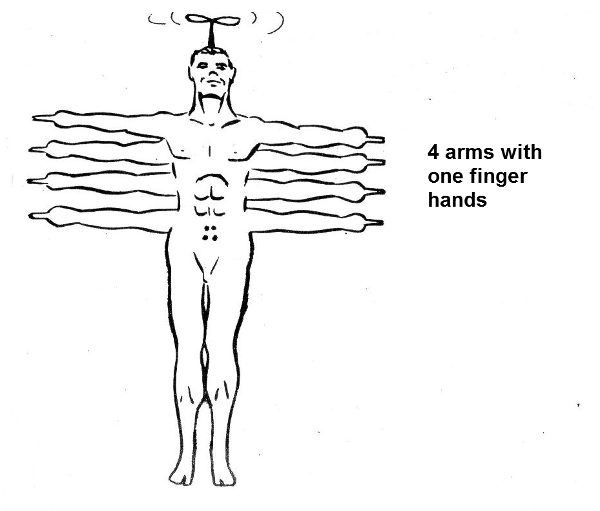Figure 2

The reader will then realize that counting objects the way we do would be perfectly possible, changing only the way of representing.

If we have a single object, it will be represented by a raised finger, as shown in Figure 3.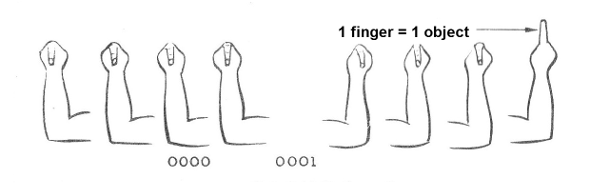Figure 3

If we have 2 objects, this finger will no longer fit. We then use the following artifice: for the individual with one finger in each hand, 2 units represent two full hands.

The representation will then be as shown in Figure 4.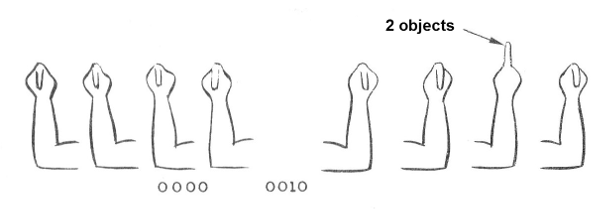Figure 4

We have one hand with the raised finger and the other with no finger, that is, 10.

If we have three objects, the representation will be as shown in Figure 5.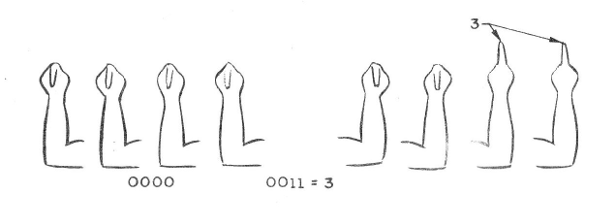Figure 5

We will have a hand that represents two units and the other one that represents one more, that is, 11.

For four objects, the problems are solved in the following way: we have 1 hand which represents that we have two times two units, that is, it is erected the finger with weight 4, as shown in Figure 6.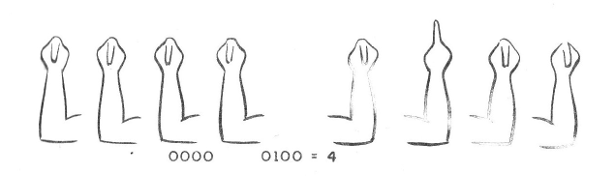Figure 6

The result is that 4 is represented as 100.

See, then, that each hand has a weight that is a power of 2. See Figure 7.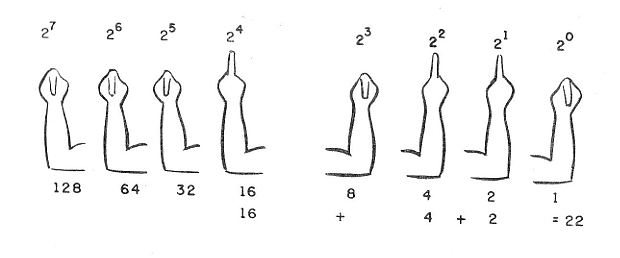Figure 7

The right hand represents the unit, that is, 20 = 1 and has this weight. The next, to the left, has weight 21 = 1 and therefore 2. The third, to the right, 22 = 4, the next, 23 = 8, coming after 24 = 16, 225 = 32, 26 = 64, to the last left 27 = 128.

See then that, the 8-arm individual of a finger can easily represent numbers up to 255 (see Figure 8).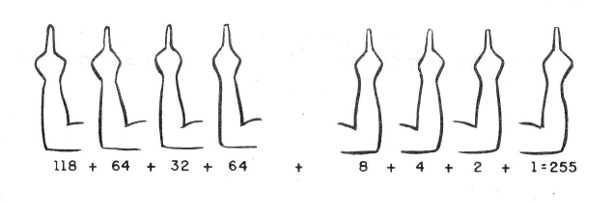Figure 8

The representation of any number of this form can then be made simply by a factorial decomposition of 2, as we will explain below.

1. Conversion to Base 2

To convert a number to base 2, whether it is in base 10, or decimal system, the procedure is simple.

It is very important for the reader to know how to do this, because as we will see, the digital circuits all work on base 2.

To make it easier, let's take the following example: we want to convert 278 to base 10 (we write 27810) into a number in base 2 (X2),

We then make the following successive division by 2: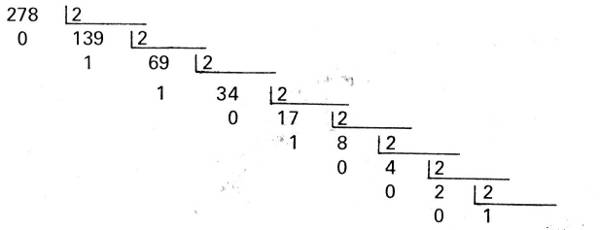Then we take the remains, starting with 1, and write in the reverse order:

100010110 = 278

Indeed:

1 X 256 = 256

0 X 128 = 0

0 X 64 = 0

0 X 32 = O

1 X 16 = 16

0 X 8 = 0

1 X 4 = 4

1 X 2 = 2

0 X 1 = O

Notice that in the binary representation, as in decimal, the digit that has the largest value is left and the one with the smallest value, but to the right.

It is customary to represent the smaler by LSD (Less Significant Digit) and the one of greater value by MSD (Most Signilicant Digit).

These terms appear a lot in digital electronics.

2. Conversion to Base 10

The conversion of a pure binary number, as we have seen, to a number in the base 10 is quite simple, just remembering that each successive number from the right to the left is double the weight of what precedes it.

So, just write the number vertically and proceed to the following calculation where we take as an example the value 11001011:

1 X 128 = 128

1 X 64 = 64

0 x 32 = o

0 X 16 = 0

1 X 8 = 8

0 X 4 = 0

1 X 2 = 2

1 X 1 = 1

Somando: = 203

Thus, 110010112 = 20310

It will be interesting for the reader to train a little by performing the following conversions:

A) Convert into pure binary:

1) 324 2) 1067 3) 1089

B) Convert into decimal:

1) 110011 2) 1101111 3) 100000 4) 1000101 5) 100101111

A) 1) 110011 2) 1101111 3) 1000101

B) 1) 51 2) 207 3) 32 4) 69 5) 303

Note that the example we take of the 8-handed individual limits the count to 255, but with more digits there is no limit to the values represented.

3. Why Binary?

The use of binary code in digital electronic circuits offers numerous advantages over a possible 10 base code.

Using the base 2 the operations are simpler than in relation to the base 10.

In an electronic circuit, on the other hand, if we were to represent the numbers from 0 to 1, we would have to have 10 levels of current or voltage.

In base 2 we can have only the presence, or the absence of tension.

Aimed at operations, readers must remember the difficulties in memorizing multiplication operations involving all figures in the form of tables.

For the multiplication, starting from the table of 2 to 9 (7 tables) we have to memorize 70 operations, that is, the value of 70 products to be able to perform any multiplication.

Working on base 2 we only memorize 4 multiplication operations:

0 x 0 = 0

0 X 1 = 0

1 X 0 = 0

1 X 1 = 1

The realization of a multiplication in binary does not differ in anything from a multiplication in decimal, as follows in the example 13 X 29.

In binary:

11101 (29)

X 1101 (13)

11101

00000

11101

11101

101111001 (377)

In the sum itself, used in multiplication the rules are also only 4:

0 + 0 = 0

0 + 1 = 1

1 + 0 = 1

1 + 1 = 1 (goes one)

From hundreds of operations that must be memorized for the operation with decimals, we fall into a much smaller number, 4 for multiplication and 4 for the sum that must be known in the calculation with binaries.

The British mathematician George Simon Boole developed at the end of the seventeenth century a mathematics that worked exclusively with the base 2. Boole assumed from the principle that two facts only admitted two types of interpretation: they were either false or true.

Taking this to the electronics we can say that a circuit can only be in two states: on or off, which corresponds precisely to base 2.

Boolean algebra was forgotten for years, being studied only as mathematical curiosity, until the electronics were developed to the point of making a practical application possible for what was then only in theory.

Then came the digital logic circuits that could be designed exactly on the basis of that Boolean algebra, performing mathematical operations most diverse, totally in function of the affirmation that the facts only admit two possible affirmations: false or true.

In Figure 9 we have a possible representation for electronic (or electrical) circuits.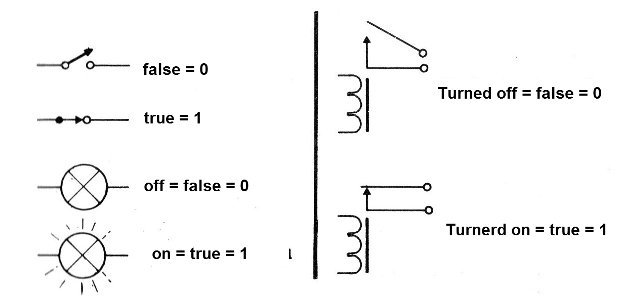Figure 9

A connected relay (closed switch) represents a true fact or a binary "1". A relay disabled (open switch) represents a false fact or one “0”.

Going to another circuit, as shown in Figure 10, we have another possibility.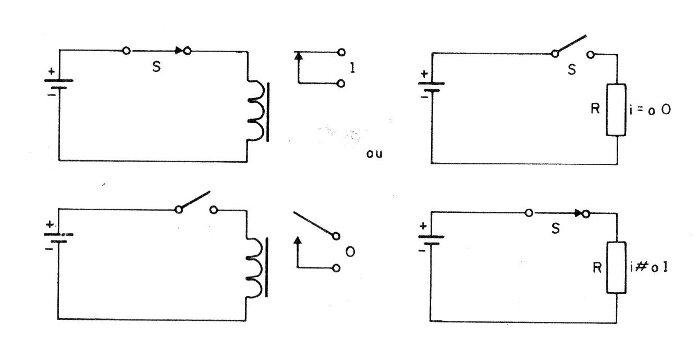Figure 10

The closed switch causes a voltage to appear on the load resistor which means the fact is true, or 1. We can also say that the voltage level is high, abbreviated by HI.

The disconnected switch represents a false fact and, therefore, the absence of voltage in the resistor. The voltage is low or zero, which can be written as LO.

This means that we have a positive logic when:

We represent the presence of voltage (or current) by 1 or HI.

We represent the absence of voltage (or current) by 0 or by LO.

We can have a logic of the opposite, or negative, that also works, when we represent the presence of voltage by 0 or LO and the absence of current by 1 or HI.

For didactic purposes, from now on we will only speak of positive logic.

Finally, the advantages of torque become apparent if we want to design a complex circuit that performs many operations with many numbers.

Each number can easily be represented by only two signal levels (0 or 1) and operations can be easily performed with few rules (which in the bottom represent few components).

The reader can imagine the difficulty in terms of a digital circuit in the base 10, trying to represent the digits from 0 to 9 through 9 voltage levels.

A small variation of the source voltage and everything will be uncontrolled: a 9 can suddenly become an 8, and everything gets complicated.

With the presence or absence of voltage, even with voltage variations, the circuits can still easily differentiate between presence and absence in a good range of values, and it remains 0, just as 1 remains 1.

With the use of a binary language, the security of the operation is much greater!

How to combine elements of a circuit to perform operations is what we will see next.

It is here to define what is a logic circuit: a logic circuit is composed of inputs and outputs that maintains a certain well defined relation.

Revised 2017